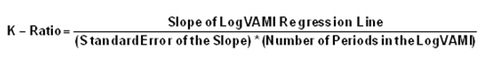# K-Ratio

Posted in Finance, Accounting and Economics Terms, Total Reads: 788

## Definition: K-Ratio

K-ratio is used to measure the performance of an equity by measuring it’s return with respect to its risk. It also acts as an indicator about how consistent stock’s return has been so far. The data required to compute this ratio is taken from VAMI(Value Added Monthly Index).

It can be Calculated as followsLars Kestner who was a statistician and also a trader in derivatives market, came up with this ratio. To calculate the ratio one has to run linear regression on log-VAMI curve. Slope of the curve represents the return and the standard error of the slope is equivalent to risk of the stock. This is a great tool the look at the risk return profile and hence the performance of any equity.

Browse the definition and meaning of more terms similar to K-Ratio. The Management Dictionary covers over 7000 business concepts from 6 categories.

Search & Explore : Management Dictionary

Similar Definitions from same Category: